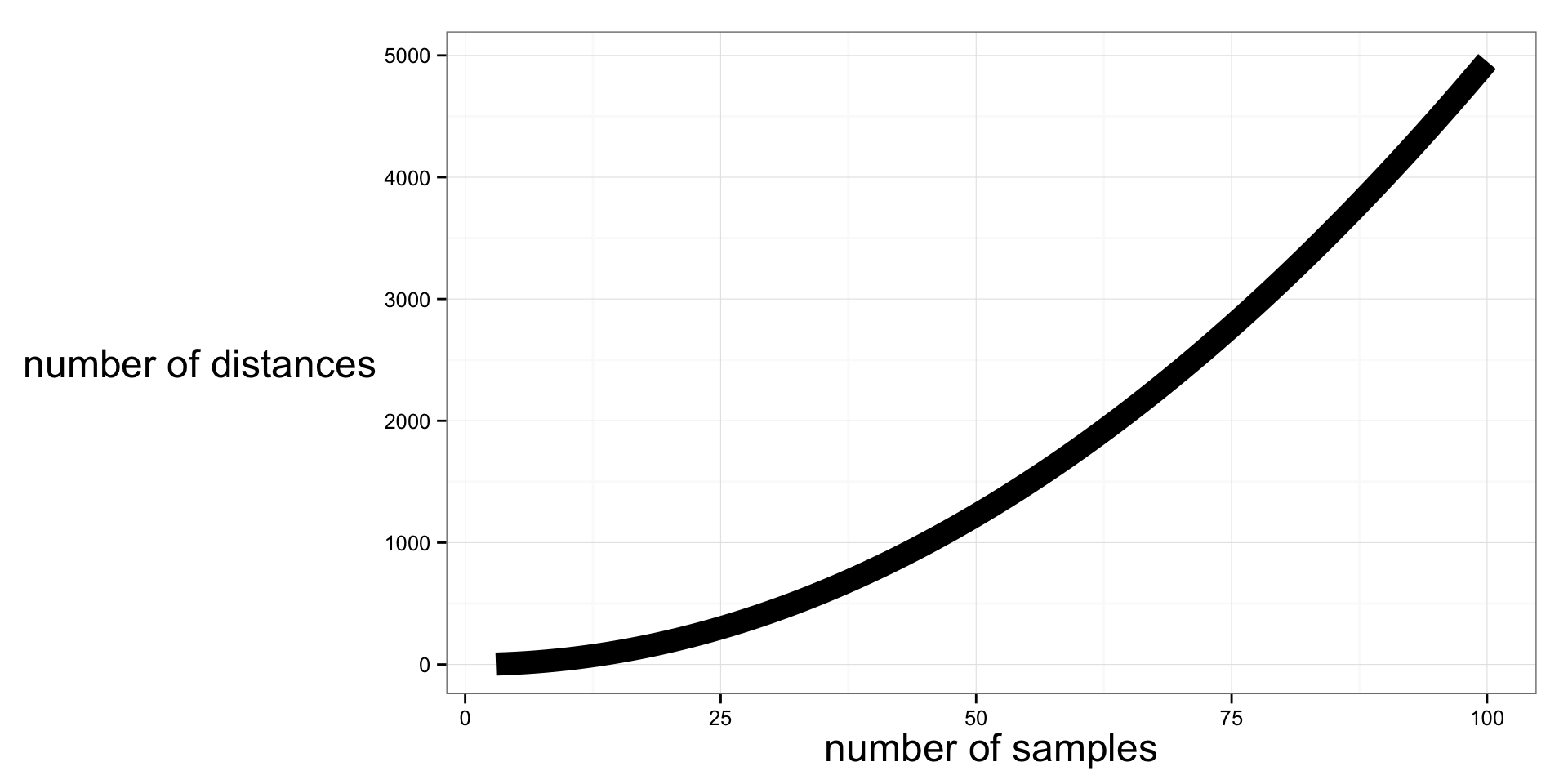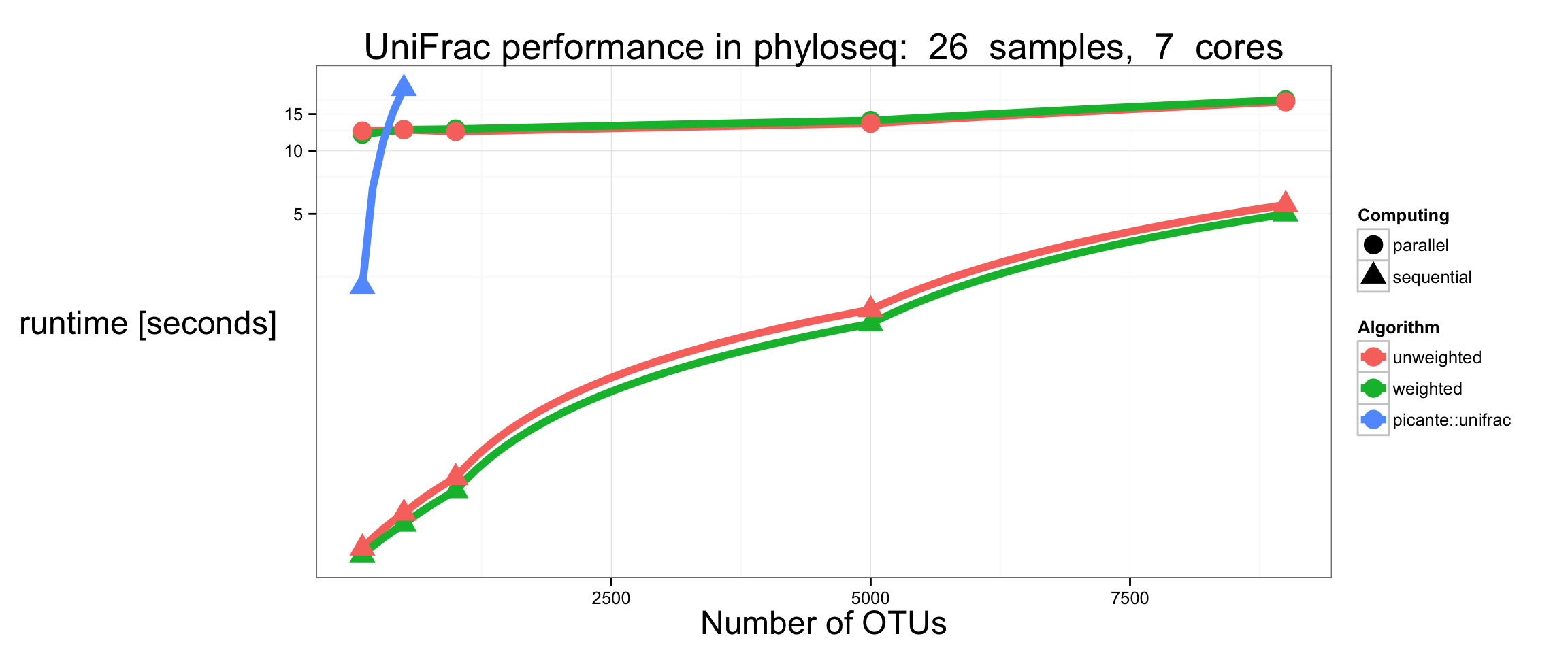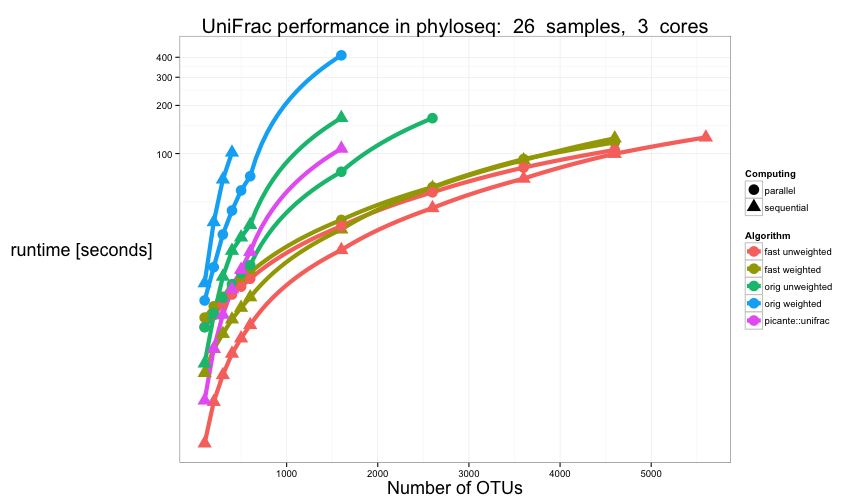# Fast Parallel UniFrac (in R)

## Background

A common tool in microbial ecology studies involving many samples is to calculate the “UniFrac” distance between all pairs of samples, and then perform various analyses on the resulting distance matrix.

The phyloseq package includes a native R implementation of the better, faster, cleaner Fast UniFrac algorithm.

There are also two very different types of the standard UniFrac calculation:

Weighted UniFrac - which does take into account differences in abundance of taxa between samples, but takes longer to calculate; and

Unweighted UniFrac - which only considers the presence/absence of taxa between sample pairs.

Both weighted and unweighted UniFrac are included in phyloseq, and all UniFrac calculations have the option of running “in parallel”] for faster results on computers that have multiple cores/processors available.

Parallelization in [the phyloseq package] is achieved using foreach loops, which flexibly support a large variety of “parallel backends” – from dual-core CPUs to large computing clusters. See foreach description in the high performance computing task view for further details, and supported architectures.

library("phyloseq"); packageVersion("phyloseq")
##  '1.9.11'
library("ggplot2"); packageVersion("ggplot2")
##  '1.0.0'
library("doParallel"); packageVersion("doParallel")
##  '1.0.8'
library("foreach"); packageVersion("foreach")
##  '1.4.2'

Set random number generator (RNG) seed, for perfect reproducibility.

set.seed(711)

Define a default theme for ggplot graphics.

theme_set(theme_bw())
fontsize = 18L
theme_update(axis.title.x = element_text(size=fontsize))
theme_update(axis.title.y = element_text(size=fontsize))
theme_update(plot.title = element_text(size=fontsize+2))

## Examples

In phyloseq, all variants of the UniFrac distance can be called with the same generic function, UniFrac. We can start with a minimal example using the esophagus example dataset.

data(esophagus)
UniFrac(esophagus, weighted=TRUE, normalized=TRUE, parallel=FALSE, fast=TRUE)
##        B      C
## C 0.2035
## D 0.2603 0.2477
(y <- UniFrac(esophagus, TRUE))
##        B      C
## C 0.2035
## D 0.2603 0.2477
UniFrac(esophagus, TRUE, FALSE)
##        B      C
## C 0.1050
## D 0.1401 0.1422
UniFrac(esophagus, FALSE)
##        B      C
## C 0.5176
## D 0.5182 0.5422

where esophagus is a small example of a "phyloseq-class" experiment-level object, imported or constructed by the phyloseq package.

If you want to compare with the unweighted-unifrac available in picante

picante::unifrac(as(t(otu_table(esophagus)), "matrix"), phy_tree(esophagus))
##        B      C
## C 0.5176
## D 0.5182 0.5422

## Performance Comparison

In the previous example, the dataset was too small to warrant parallel computing. In fact, the computational overhead to perform the calculation with multiple processors would probably have been more expensive (take longer) than just calculating the 3 distance values sequentially. However, as the numbers of samples and OTUs in a dataset increases, the increased speed of computing these distances in parallel becomes very worthwhile, as shown in the following demonstration.

data("GlobalPatterns")
GP = prune_taxa(taxa_sums(GlobalPatterns)>0, GlobalPatterns)
GP
## phyloseq-class experiment-level object
## otu_table()   OTU Table:         [ 18988 taxa and 26 samples ]
## sample_data() Sample Data:       [ 26 samples by 7 sample variables ]
## tax_table()   Taxonomy Table:    [ 18988 taxa by 7 taxonomic ranks ]
## phy_tree()    Phylogenetic Tree: [ 18988 tips and 18987 internal nodes ]
choose(nsamples(GP), 2)
##  325

The GP example dataset has 26 samples and 18988 OTUs. Each distance in a UniFrac result matrix represents the pairwise distance between samples. The greater the number of samples to compare, the larger the number of pairwise distances to calculate. That is, there are $${n \choose 2}$$ combinations, in this case 325 pairwise distances that will be calculated by UniFrac on the GP dataset. Because the value of one distance does not depend on another, this becomes “embarassingly parallelizable” for a dataset with a large number of samples, or for which each pair of samples takes a noticeable amount of time to compute.

### Distance combinations

To understand this graphically, let’s make a simple plot of the number of pairwise distance elements that must be calculated as a function of the number of samples

nsamples = 3:100
ndistances = sapply(nsamples, choose, 2)
p = ggplot(data.frame(nsamples, ndistances), aes(nsamples, ndistances)) + geom_line(size=5)
p = p + xlab("number of samples") + ylab("number of distances")
p### Computing Time Example

The following code first computes and then plots the computation time required to calculate the distance matrix for the GP dataset at varying numbers of OTUs. As you can see, for datasets with less than 1000 OTUs, the choice of algorithm has only inconsequential effect on computation time, with all approaches returning a result in under 10 seconds. However as more and more taxa are included (more complex phylogenetic tree) in the dataset, the computation time increases exponentially; and at a much faster rate for variants of the original UniFrac algorithm. As pointed out in the article describing Fast UniFrac, there is much less difference in performance between unweighted/weighted Fast UniFrac, making weighted UniFrac distances much more accessible (than previously) for large datasets.

#### Parameters

save.data.name = "unifrac.RData"

Threshold, in seconds. The maximum allowed time for one trial. Trials will not be conducted for that argument combination after this amount of time…

threshold = 15

Number of cores to use for parallel trials

Ncores = 7

Create a vector of the numbers of taxa, Ntaxa, and then create a list of phyloseq objects for each one. Will loop on the list. Define vector of the number of OTUs to include.

Ntaxa <- c(100L, 500L, seq(1000L, 10000, 4000))
Ntaxa
##   100  500 1000 5000 9000
physeq_list <- vector("list", length(Ntaxa))
names(physeq_list) <- as.character(Ntaxa)

Create a list with of random subset with N OTUs

for( N in Ntaxa ){
# Randomly subset N taxa from the original
ex   <- prune_taxa(sample(taxa_names(GP), N), GP)
# Assign a random root from the sub-sampled dataset
phy_tree(ex) <- ape::root(phy_tree(ex), sample(taxa_names(ex), 1), resolve.root=TRUE)
# Add to the list of physeq objects
physeq_list[[as(N, "character")]] <- phyloseq(otu_table(ex), phy_tree(ex))
}

Create table of combinations of function calls

UFcalls <- expand.grid(
parallel=c(TRUE, FALSE),
weighted=c(TRUE, FALSE),
KEEP.OUT.ATTRS=FALSE)

The following creates a list appropriate for do.call(): UFcalls[1, , drop=TRUE]

Initialize data.frame of results

time.frame <- data.frame(matrix(NA, 0, 5, FALSE))

StillOK is index for keeping track of which call combinations in UFcalls are still below time threshold

stillOK <- rep(TRUE, nrow(UFcalls))

Loop over each incrementally more-complex data subset. Stop tabulating a combination if run-time gets above a threshold. For each element of physeq_list, track the time for each function call indicated by UFcalls.

for( N in Ntaxa ){
if( sum(stillOK) > 0 ){
for( i in which(stillOK) ){ # i<-1
# Create list of arguments to UniFrac
call.list <- c(physeq=physeq_list[[as.character(N)]], UFcalls[i, , drop=TRUE] )
# Register the appropriate parallel or sequential backend
if( call.list$parallel){ registerDoParallel(makeCluster(Ncores)) } else { registerDoSEQ() } # Build, and call UniFrac with list of arguments runtime <- system.time(do.call("UniFrac", call.list))["elapsed"] # Store the values in growing data.frame time.frame <- rbind(time.frame, data.frame(taxa=N, runtime, UFcalls[i, , drop=TRUE]) ) # Check if this runtime was below threshold, if not, mark it if( runtime > threshold ){ # mark the combination as too long stillOK[i] <- FALSE } } } } Now run one more loop for picante::unifrac() time (unweighted, not-parallel). Initialize picante. picante.frame <- data.frame(matrix(NA, 0, 2, FALSE)) stillOK <- TRUE picante::unifrac loop over each N in Ntaxa. for( N in Ntaxa ){ if( stillOK > 0 ){ # Create list of arguments to UniFrac call.list <- c(physeq=physeq_list[[as.character(N)]], UFcalls[i, , drop=TRUE] ) # Determine if should register parallel backend or sequential if( call.list$parallel){
registerDoParallel(makeCluster(Ncores))
}
# calculate, score run-time
exOTU <- as( t(otu_table(physeq_list[[as.character(N)]])), "matrix")
extre <- phy_tree(physeq_list[[as.character(N)]])
runtime <- system.time( picante::unifrac(exOTU, extre) )["elapsed"]
# Store the values in growing data.frame
picante.frame <- rbind(picante.frame, data.frame(taxa=N, runtime) )
if( runtime > threshold ){
# If this runtime was above threshold, mark it and stop loop
stillOK <- FALSE
}
}
}

That part takes a long time. Save the data before plotting. Plotting is quick

save.image(save.data.name, compress="bzip2")

#### Now build final data.frame of results

Modify logical parameter values to informative text factor

time.frame[,"weighted"] <- factor(ifelse(time.frame[,"weighted"], "weighted", "unweighted"))
time.frame[,"parallel"] <- factor(ifelse(time.frame[,"parallel"], "parallel", "sequential"))

Add an “Algorithm” column that summarizes the algorithm approach.

time.frame.plot <- data.frame(time.frame, algorithm=time.frame[, "weighted"] )

Add picante.frame to time.frame

picante.frame.plot <- data.frame(picante.frame, parallel="sequential", weighted="unweighted", algorithm="picante::unifrac")

Finally, create a new separate, everything-combined data.frame for plotting

time.df <- rbind(time.frame.plot, picante.frame.plot)

#### Now build ggplot of results.

p = ggplot(time.df, aes(taxa, runtime, color=algorithm, shape=parallel))
p = p + geom_path(size=2) + geom_point(size=5)
p = p + ggtitle(paste("UniFrac performance in phyloseq: ", nsamples(ex), " samples, ", Ncores, " cores"))
p = p + scale_shape_discrete(name="Computing") + scale_colour_discrete(name="Algorithm")
p = p + ylab("runtime [seconds]") + xlab("Number of OTUs")

Before plotting, transform the vertical coordinate system to log10. See the documentation for coord_trans for more details.

p = p + coord_trans(y = "log10")

Now actually “print” the graphic

pLegend Computation time required to calculate the distance matrix for a 26-sample experiment at varying numbers of taxa. For comparison, the performance of the original unweighted UniFrac algorithm as implemented in the “picante” package is also included.

Save the workspace for later.

save.image(save.data.name, compress="bzip2")

# Old-Version Time Trial Results

In case you are curious, phyloseq 1.9.11+ switched to using low-level C code for certain “botteleneck” steps in the Fast UniFrac algorithm. The old time trial is shown here for comparison.Legend Previous time trial comparison results. Note how much faster the new version is, and in the newer case, how much larger the dataset is needed for the overhead cost of initializing parallelization becomes worthwhile.